# 12 6. In a nuclear reaction carbon nucleus, C collides elastically in a head on collision...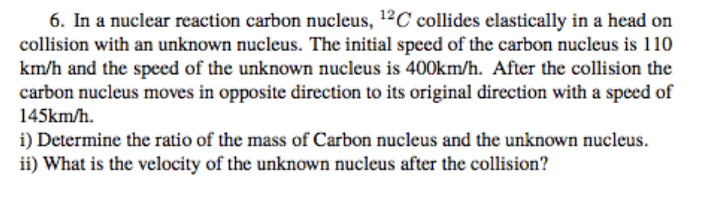12 6. In a nuclear reaction carbon nucleus, C collides elastically in a head on collision with an unknown nucleus. The initial speed of the carbon nucleus is 110 km/h and the speed of the unknown nucleus is 400km/h. After the collision the carbon nucleus moves in opposite direction to its original direction with a speed of 145km/h. i) Determine the ratio of the mass of Carbon nucleus and the unknown nucleus. ii) What is the velocity of the unknown nucleus after the collision?

mass of carbon = m1

mass of unknown = m2

before collision

speed of m1 , v1i = 110 km/h

speed of m2 , v2i = -400 km/h

after collision

speed of m1 , v1if = -145 km/h

speed of m2 , v2f = 0 km/h

from momentum conservation

initial momentum before collision

Pi = m1*v1i + m2*v2i

after collision final momentum

Pf = m1*v1f + m2*v2f

from momentum conservation

total momentum is conserved

Pf = Pi

m1*v1i + m2*v2i = m1*v1f + m2*v2f .....(1)

from energy conservation

total kinetic energy before collision = total kinetic energy after collision

KEi = 0.5*m1*v1i^2 + 0.5*m2*v2i^2

KEf =   0.5*m1*v1f^2 + 0.5*m2*v2f^2

KEi = KEf

0.5*m1*v1i^2 + 0.5*m2*v2i^2 = 0.5*m1*v1f^2 + 0.5*m2*v2f^2 .....(2)

solving 1&2

we get

v1f = ((m1-m2)*v1i - (2*m2*v2i))/(m1+m2)

-145 = ((m1 - m2)*110 - (2*m2*400))/(m1 + m2)

-145*m1 - 145*m2 = 110*m1 - 110*m2 - 800*m2

m1*(110 + 145 ) = m2*(800 - 145 + 110)

v2f = ((m2-m1)*v2i + (2*m1*v1i))/(m1+m2)

v2f = (-(m2-3m2)*400 +(2*3m2*110))/(3m2 + m2)

v2f = (800 + 660)/4

#### Earn Coin

Coins can be redeemed for fabulous gifts.

Similar Homework Help Questions
• ### A proton (mass 1 u) moving at 9.00 10^(6) m/s collides elastically and head-on with a...

A proton (mass 1 u) moving at 9.00 10^(6) m/s collides elastically and head-on with a second particle moving in the opposite direction at 2.00 10^(6) m/s. After the collision, the proton is moving opposite to its initial direction at 5.40 10^(6) m/s. Find the mass and final velocity of the second particle. [Take the proton's initial velocity to be in the positive direction.]

• ### A neutron in a nuclear reactor makes an elastic, head-on collision with the nucleus of a...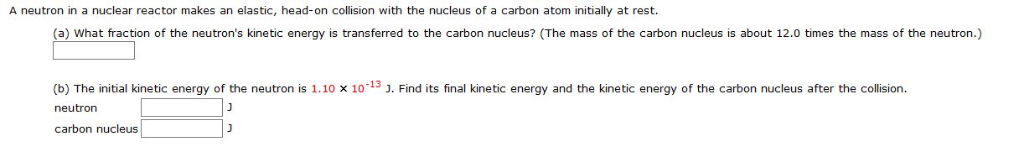A neutron in a nuclear reactor makes an elastic, head-on collision with the nucleus of a carbon atom initially at rest. A neutron in a nuclear reactor makes an elastic, head-on collision with the nucleus of a carbon atom initially at rest. (a) What fraction of the neutron's kinetic energy is transferred to the carbon nucleus? (The mass of the carbon nucleus is about 12.0 times the mass of the neutron.) (b) The initial kinetic energy of the neutron is...

• ### A neutron in a nuclear reactor makes an elastic, head-on collision with the nucleus of a...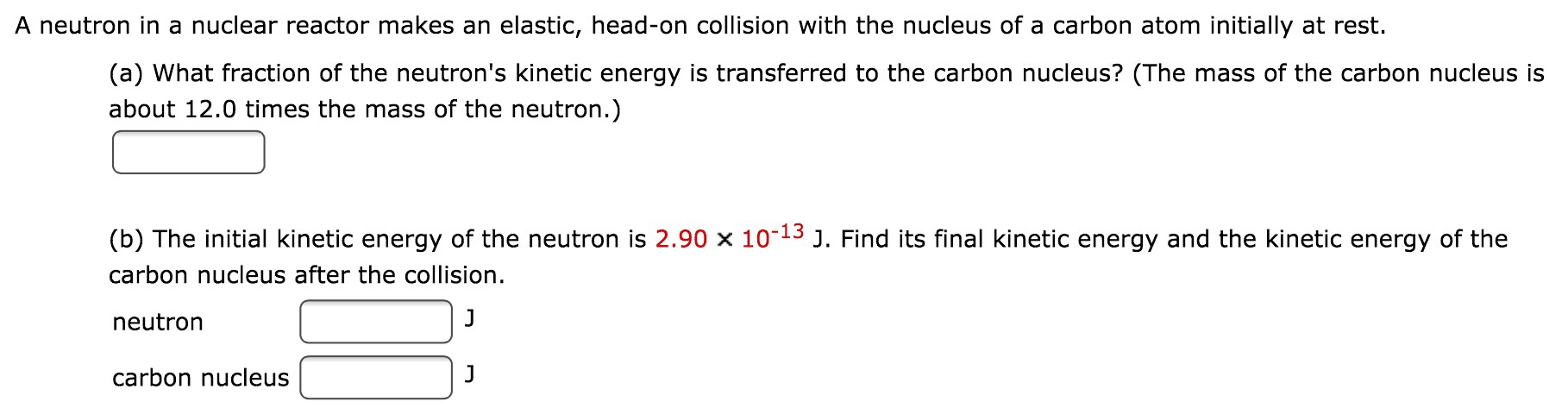A neutron in a nuclear reactor makes an elastic, head-on collision with the nucleus of a carbon atom initially at rest. (a) What fraction of the neutron's kinetic energy is transferred to the carbon nucleus? (The mass of the carbon nucleus is about 12.0 times the mass of the neutron.) (b) The initial kinetic energy of the neutron is 2.90 X 10^-13 J. Find its final kinetic energy and the kinetic energy of the carbon nucleus after the collision. neutron...

• ### A proton moving at v1 = 7.9Mm/s collides elastically and head-on with a second proton moving...

A proton moving at v1 = 7.9Mm/s collides elastically and head-on with a second proton moving in the opposite direction at v2 = 8.6Mm/s. Choose positive velocities in the direction of v? 1. a. Find the velocity of the first proton after the collision b. Find the velocity of the second proton after the collision.

• ### A neutron collides elastically with a helium nucleus (at rest initially) whose mass is four times...

A neutron collides elastically with a helium nucleus (at rest initially) whose mass is four times that of the neutron. The helium nucleus is observed to rebound at an angle x = 43° from the neutron's initial direction. The neutron's initial speed is 6.4*10^5 m/s. Determine the angle at which the neutron rebounds, measured from its initial direction. I have been trying to answer this question for days. Below are the answers I have tried plugging in, but it was...

• ### A billiard ball collides in an elastic head-on collision with a second stationary identical ball. After...

A billiard ball collides in an elastic head-on collision with a second stationary identical ball. After the collision which of the following conditions applies to the first ball? A) maintains the same velocity as before B)has one half its initial velocity C)comes to rest D)moves in the opposite direction E)doubles its initial velocity

• ### 10) A proton moving at 2.44x105 m/s elastically collides with a helium nucleus that is initially...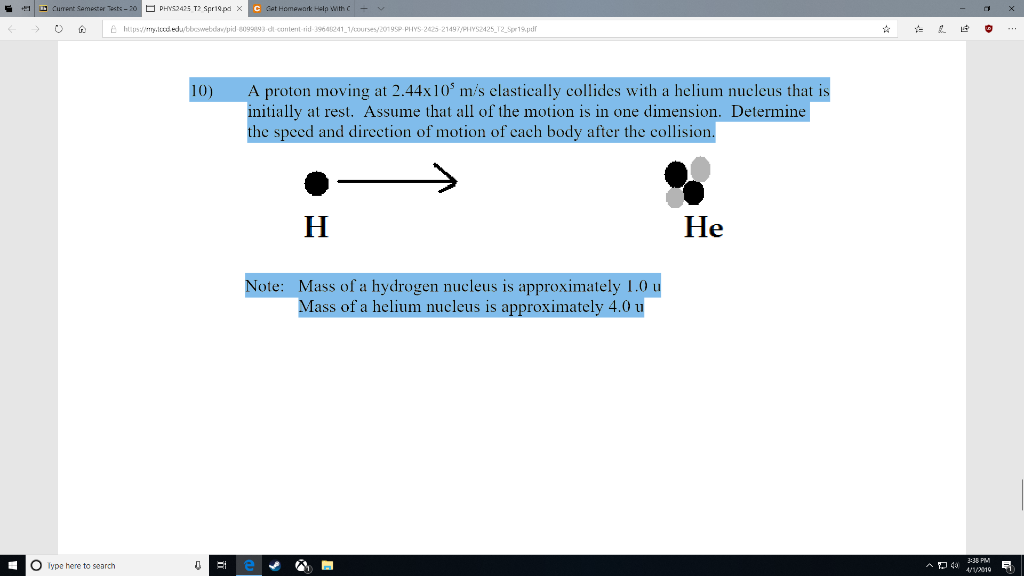10) A proton moving at 2.44x105 m/s elastically collides with a helium nucleus that is initially at rest. Assume that all of the motion is in one dimension. Determine the speed and direction of motion of each body after the collision. Note: Mass of a hydrogen nucleus is approximately 1.0 u Mass of a helium nucleus is approximately 4.0 u Please post both the equation and the final answer. thank you 10) A proton moving at 2.44x 103 m/s clastically...

• ### A neutron collides elastically with a helium nucleus (at rest initially) whose mass is four times...

A neutron collides elastically with a helium nucleus (at rest initially) whose mass is four times that of the neutron. The helium nucleus is observed to move off at an angle θ′2=45∘. The neutron's initial speed is 6.0×105 m/s . Determine the angle of the neutron, θ′1, after the collision. Determine the speeds of the two particles, v′n and v′He, after the collision

• ### 3. A neutron of mass m and speed v collides elastically with a carbon nucleus of...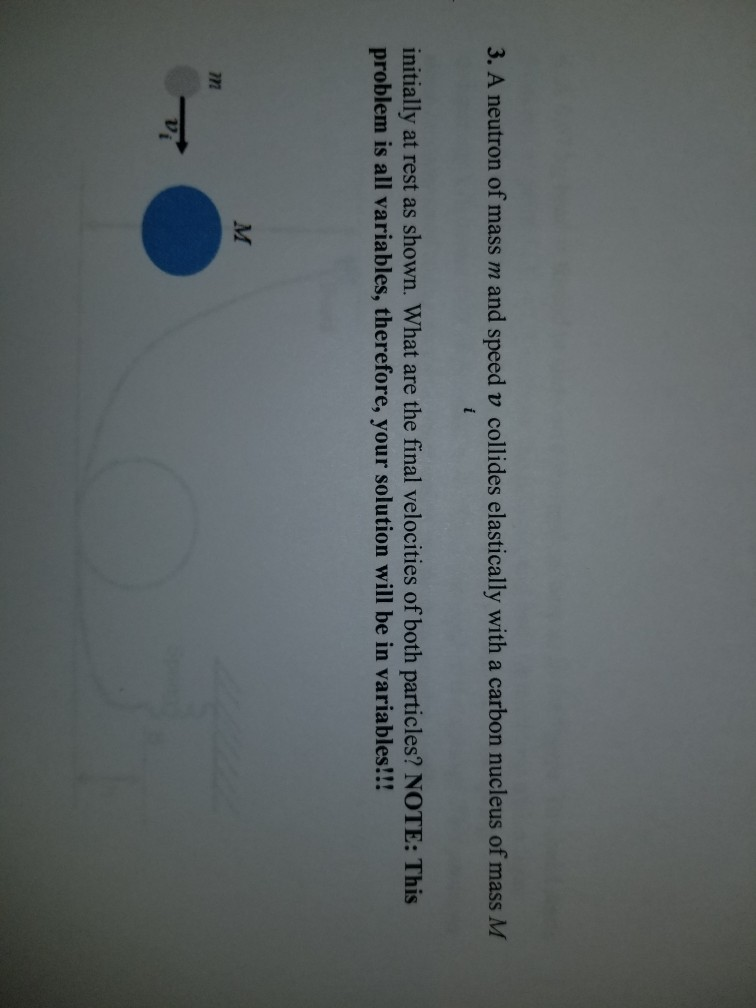3. A neutron of mass m and speed v collides elastically with a carbon nucleus of mass M initially at rest as shown. What are the final velocities of both particles? NOTE: This problem is all variables, therefore, your solution will be in variables!!! M Vi

• ### On a frictionless surface. a block of mass M moving at speed v collides elastically with...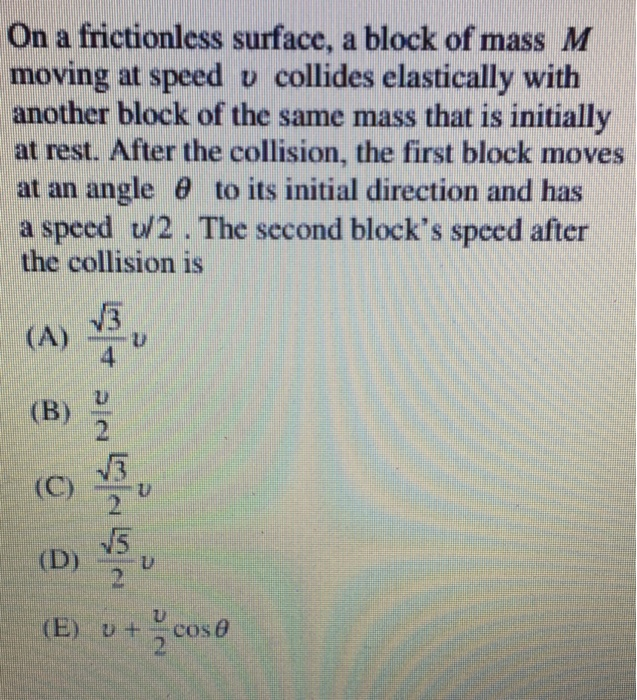On a frictionless surface. a block of mass M moving at speed v collides elastically with another block of the same mass that is initially at rest. After the collision, the first block moves at an angle θ to its initial direction and has a speed U/2. The second block's speed after the collision is 3 4 (B) 2 2 U) (E) ut cose

Free Homework Help App